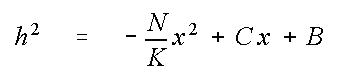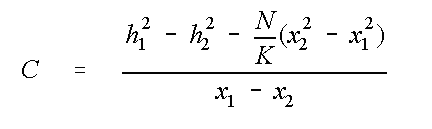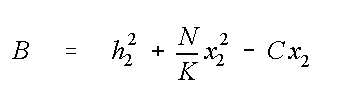# EPA On-line Tools for Site Assessment Calculation

 In Module Home Objectives Table of Contents Previous < Next >

 15 of 67

#### Theory

The recharge calculator is based upon two solutions of the one-dimensional Dupuit equation for flow in unconfined aquifers. These solutions are combined to determine the position of the phreatic surface (water table) and a streamline originating at the water table, the gradient, ground water fluxes and travel times for sorbing contaminants. The following sections outline the methods used for calculating these quantities.

#### Phreatic Surface

The first solution is used to determine the location of the phreatic surface Bear (1972, page 379) and is given bywhere h is the elevation of the phreatic surface above the datum (bottom of the aquifer) [L], N is the recharge rate [L3/L2/T], K is the hydraulic conductivity of the aquifer [L/T], x is the distance [L]. The constants C and B are determined fromandwhere x1 and x2 [L] are up and down gradient locations in the aquifer, and h1 and h2 [L] are the corresponding phreatic surface elevations or heads.

Bear's solution does not require that the water table elevation decrease monotonically downgradient. Depending on the values input for recharge, hydraulic conductivity and heads, there could be a ground water divide between the two specified end points.

 Previous Top ^ Next

Home | Glossary | Notation | Links | References | Calculators

Contact Jim Weaver to ask a technical question on this material.